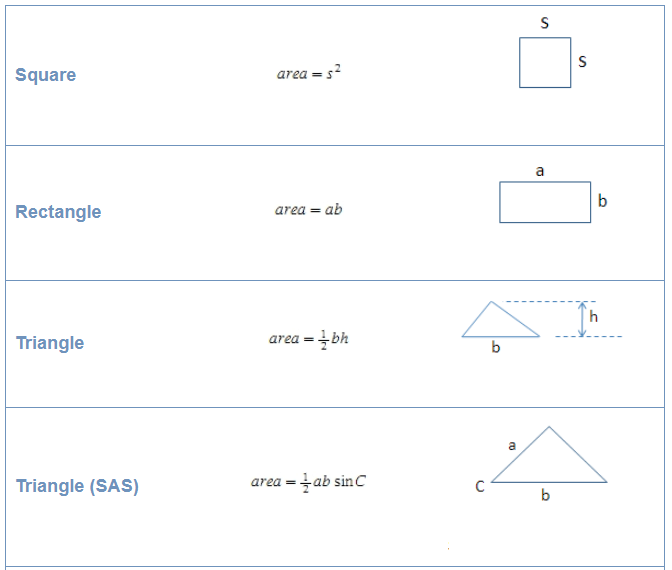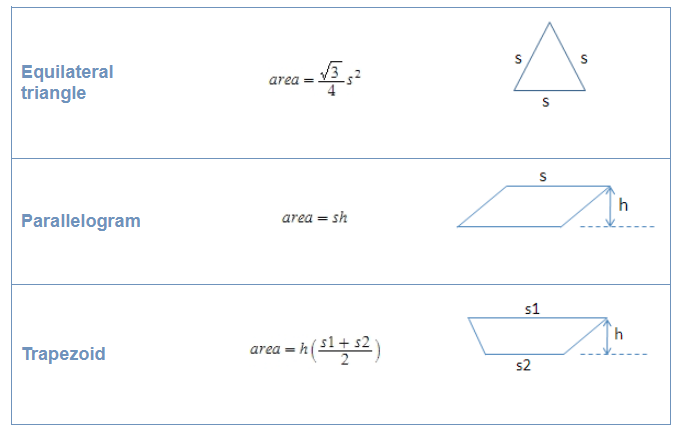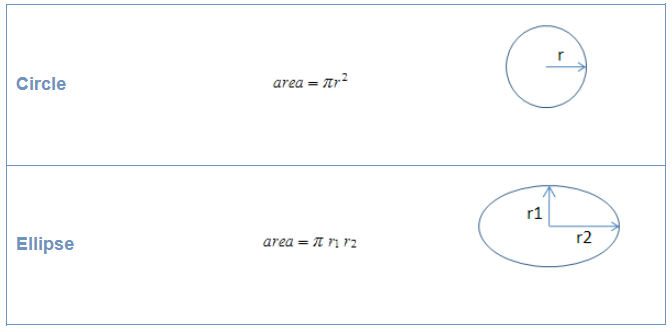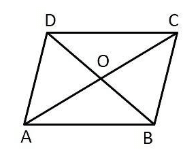# AP Board Class 9 Maths Chapter 11 Areas

AP Board Class 9 Maths Chapter 11, which covers the topic of areas has been designed to help students learn how to calculate the areas of triangles, squares, rectangles and quadrilaterals by using some formulae.  In this unit, they will also explore the basis of these formulae and discuss how are they derived. AP Board Class 9 Maths Chapter 11 Areas explain the concepts using simple language and easy sample exercises. Students can refer these and prepare for the exams.

## What is Area?

In simple terms, the area of a shape or a figure is a measurement of how much space is available on a flat surface or how much plane is enclosed by a certain figure. As there are several types of shapes there are different ways to find the area for each shape. Area is measured in square units.

### Areas of Shapes and Their Formulas

The area of shapes can be found using some formulas given below;Students can check out some of the chapter questions along with their solutions to know more about the concept.

Question 1: Show that the diagonals of a parallelogram divide it into four triangles of equal area.

Solution:

Consider a parallelogram ABCD as shown belowAs seen, the diagonals BD and CA bisect each otherat the point O.

O is the midpoint of BD, hence OD = OB

Similarly, O is the midpoint of AC, hence OC = OA

In △ ABC,

As OC = OA, OB is the median of △ ABC,

Therefore, the area of △ BOA = the area of △ BOC……..(1)

As OC = OA, OD is the median of △ ADC

Therefore, the area of △ DOA = the area of △ DOC……….(2)

Median divides the triangle into two equal areas

Similarly, in △ ADB since OD = OB, OA is the median

Hence, the area of △ AOB = the area of △ AOD…………….(3)

From (1), (2) and (3) we can conclude that the diagonals of a parallelogram divide it into four triangles of equal area

Know more about Andhra Pradesh Board and access various study materials including syllabus, exam timetable, etc. at BYJU’s.